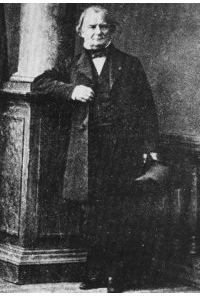# What is the Ideal Gas Law? What are Gas Laws?

## Introduction

Gases are nearly everywhere: the air you breathe into your lungs is a gas, and gas is also inside soda, the space shuttle, neon lamps, volcanoes, and the oceans, to name a few examples. Gases in your lungs, volcanoes and oceans occur naturally, but it is because we understand their behavior under certain conditions that we are able to create soda and neon lamps, and are also able to operate the space shuttle.

In previous articles, we discussed Boyle’s Law, Charles’ Law, and the Law of Gay-Lussac. These laws explained interesting effects such as why beer kegs and soda cans can explode under certain conditions. They were discovered by holding any of the variables pressure, temperature, or volume, constant with respect to the other two.

But they can also be combined, and that is where the ideal gas law comes in.

## What is the Ideal Gas Law?

The ideal gas law was discovered in 1834 by physicist and engineer Benoît Paul Émile Clapeyron (1799 -1864).Clapeyron is one of the founding fathers of thermodynamics; he was the one who gave wide exposure to the little known work of Sadi Carnot, and he had a profound influence on Lord Kelvin and Clausius. The Clausius-Clapeyron equation, which describes the slope of phase boundary lines such as those between a liquid and gas, is named after him.

The ideal gas law is an equation of state that is very important and fundamental in thermodynamics. It is found by combining the laws of Boyle, Charles, and Gay-Lussac, into one elegant equation as follows below.

For a pressure P and volume V directly proportional to a temperature T we have:

1) PV ˜ T

or

2) PV ˜ mT,

where m is the mass of the gas.

All gases do not have the same mass so to simplify matters, we would like to have a constant that is the identical for all of them. For this reason, we convert m into a proportionality constant using a mole. The concept of a mole was previously discussed in Avogadro’s Number.

Rewriting equation 2 in terms of moles, we have:

3) PV ˜ nT

or

4) PV = nRT.

R is a constant of proportionality called the universal gas constant, and in accordance with its name it is the same for all gases. Its value has been determined experimentally. In SI units of Joules and Kelvin, R is 8.315 J/(mol*K), while in BTU it is 1.99 calories/(mol*K). If we are interested in specifying volume in liters and pressure in atm, then R is 0.0821 (L*atm)/mol*K.

The ideal gas law applies for most gases in an equilibrium state where it is not too dense, and P is around atmospheric pressure. Real gases approximate these conditions enough such that the law is applicable in every day life.

In part two, we will learn how to use this law to find the mass of the total number of air particles inside your room.

## References

Physics for Scientists and Engineers by Douglas Giancoli

Fundamentals of Physics by Halliday, Resnick, and Walker

An Introduction to Thermal Physics by Daniel V. Schroeder

Image Credits

Clayperon from The Encyclopedia of the Earth

## Resources

Ideal Gas Law Simulation (More like an addictive game; Java is required)

Another Addictive Ideal Gas Simulation (Takes a while to load; Java is required; makes a cool exploding sound if you deliberately

crack the chamber)

## This post is part of the series: Introduction to the Ideal Gas Law

This series gives an elementary, non-calculus based introduction to the ideal gas law, including an account of its origins and how to use it to derive Boyle’s, Charles’, and Gay-Lussac’s Laws.